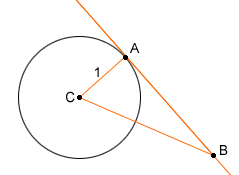Who is asking: Teacher Level : High School I am stuck on a tangent? Is there a relationship between the tangent of a curve(line touching the curve at one point) and tangent (the trigonometric function)? Thanks for your help. Hi, Sure: Start with a circle whose radius is 1 and centre is C, a point A on the circle, and a point B on the line tangent to the circle at A. Then the TANGENT of angle ACB is the length of the segment AB intercepted by that angle on the tangent line.Chris Go to Math Central